20.2: Structure and Properties of Carboxylic Acids

Objectives

After completing this section, you should be able to

1. describe the geometry and electronic structure of a simple carboxylic acid; for example, acetic acid.
2. describe the hydrogen bonding that occurs between carboxylic acid molecules, and hence account for the relatively high boiling points of these compounds.
3. write an expression for the dissociation constant of a given carboxylic acid, and use it to calculate dissociation constants, percentage dissociation, etc.
4. identify carboxylic acids as being weaker acids than mineral acids, such as hydrochloric acid, but stronger acids than alcohols.
5. use the concept of resonance to explain why carboxylic acids are stronger acids than alcohols.
6. draw an orbital picture of a carboxylate anion to show the equivalence of the two oxygen atoms.
7. explain why the two carbon‑oxygen bond lengths are identical in sodium carboxylate, but different in carboxylic acid.
8. write an equation for the reaction of a carboxylic acid with a base, such as sodium hydroxide.
Study Notes

You might wish to review Sections 2.7, “Acids and Bases: The Brønsted‑Lowry Definition” and 6.7, “Describing a Reaction: Equilibria, Rates, and Energy Changes” in conjunction with this section.

In the reading, the discussion of the role of resonance in the acidity of a carboxylic acid explains that the two carbon‑oxygen bonds in the delocalized carboxylate anion are identical (both 1.27 Å). However, in the structure of a carboxylic acid the $$\ce{C-O}$$ bond (1.20 Å) is shorter than the $$\ce{C-OH}$$ bond (1.34 Å).Structure of the carboxyl acid group

Carboxylic acids are organic compounds which incorporate a carboxyl functional group, CO2H. The name carboxyl comes from the fact that a carbonyl and a hydroxyl group are attached to the same carbon.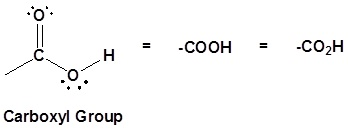The carbon and oxygen in the carbonyl are both sp2 hybridized which gives a carboxylic acid a trigonal planar shape (around the carbonyl carbon) making the bond angles roughly 120o. A resonance structure exists where one of the lone pairs of the hydroxyl oxygen (OH) is conjugated with the pi bond system of the carbonyl group. The conjugation can be represented by the resonance structure shown below which holds all the atoms in the carboxylic acid in a co-planar arrangement.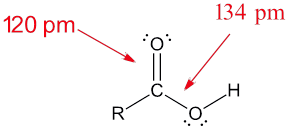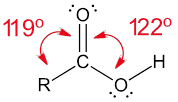The effects of conjugation in a carboxylic acid can be seen when comparing the electrostatic potential maps of ethanol and acetic acid. Conjugation creates a greater polarization in the O-H bond in acetic acid, as shown by its darker blue color, which subsequently leads to a greater acidity in carboxylic acids.Acidity of Carboxylic Acids

Carboxylic acids are named such because they tend to be more acidic than other functional groups in organic chemistry. In dilute aqueous solutions, they act as Brønsted–Lowry acids and partially dissociate to produce the corresponding carboxylate ion and hydronium (H3O+).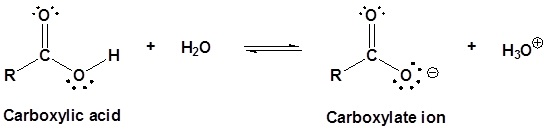The extent of this dissociation is described by the $$K_a$$ value of the carboxylic acid. In a 0.1 M solution of acetic acid ($$K_a = 1.75 \times 10^{-5}$$ @ 25 °C) only about 0.1% of the molecules are dissociated.

\begin{align*} K_{eq} &= K_a \\[4pt] &= \dfrac{[\ce{RCOO^{-}}][\ce{H3O^{+}}]}{[\ce{RCOOH}]} \\[4pt] &= 1.75 \times 10^{-5} \end{align*}

with $$pK_a = -\log K_a$$.

The pKa 's of some typical carboxylic acids are listed in Table $$\PageIndex{1}$$. The factors which affect the acidity of different carboxylic acids will be discussed in the next section of this text.

Table $$\PageIndex{1}$$: Acidity of example carboxylic acids
Compound Ka pKa
F3CCO2H 0.59 0.23
Cl3CCO2H 0.22 0.66
p-O2NC6H4CO2H 3.39 X 10-4 3.47
HCO2H 1.78 X 10-4 3.75
C6H5CO2H 6.31 X 10-5 4.20
p-CH3OC6H4CO2H 3.55 X 10-5 4.45
CH3CO2H 1.75 X 10-5 4.76
CH3CH2CH2CO2H 1.51 X 10-5 4.82

The acidity of carboxylic acids comes about, in part, due to resonance stabilization of the carboxylate conjugate base. A classic example compares the relative acidity of ethanol and acetic acid, but the conclusions we reach will be equally valid for all alcohol and carboxylic acid groups. Despite the fact that they are both oxygen acids, the pKa values of ethanol and acetic acid are very different (acetic acid is more than 1011 times more acidic than ethanol). In both species, the negative charge on the conjugate base is held by an oxygen so electronegativity does not explain the difference in acidity.For acetic acid, however, there is a key difference: a resonance contributor can be drawn in which the negative charge is localized on the second oxygen of the group. The two resonance forms for the conjugate base are equal in energy allowing for the the negative charge on the acetate ion to be equally shared between two oxygens. In the ethoxide anion, by contrast, the negative charge is ‘locked’ on the single oxygen. This stabilization leads to a marked increase in stability of the acetate anion, as illustrated by the energy diagram shown below. The increased stabilization of the acetate anion conjugate base promotes the acidity of acetic acid.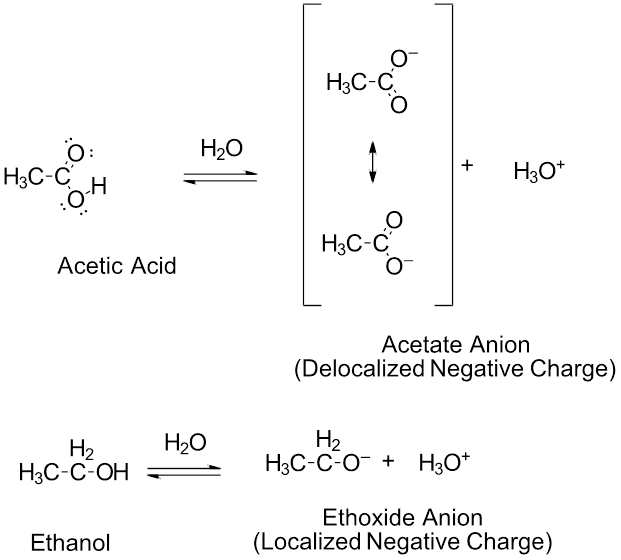The delocalization of charge by resonance has a very powerful effect on the reactivity of organic molecules, enough to account for the difference of over 11 pKa units between ethanol and acetic acid (and remember, pKa is a log expression, so we are talking about a difference of over 1011 between the acidity constants for the two molecules). The acetate anion is that much more stable than the ethoxide anion, all due to the effects of resonance delocalization.

X-ray crystallographic studies provide experimental evidence of the acetate anion resonance hybrid. Both C-O bonds in acetate are 126 pm which is roughly the average of C=O double bond (123 pm) and the C-O single bond (132 pm) of acetic acid. Both of the C-O bonds in the acetate anion are considered to have a bond order of 1 1/2. The negative charge is equally spread between the two oxygens giving them each a charge of -1/2.Resonance structures and resonance hybrid of acetate ion.

Physical Properties of Some Carboxylic Acids

Carboxylic acids tend to have higher boiling points when compared to ethers, alcohols, aldehydes, or ketones with a similar molecular weight. For example acetic acid and ethanol have boiling points of 117.9 oC and 78.3 oC respectively despite the fact that they both contain two carbons. Because carboxylic acids and alcohols both contain an O-H bond they are strongly associated by a hydrogen-bonding intermolecular force. The difference is that for carboxylic acids two molecules of a carboxylic acid form two hydrogen bonds with each other to create a cyclic dimer (pair of molecules). Two alcohol molecules can only form one hydrogen bond between each other. Thus, carboxylic acids have stronger intermolecular forces and higher boiling points than their corresponding alcohols.Table $$\PageIndex{2}$$: Intermolecular hydrogen bonding in carboxylic acids.
Formula Common Name Source IUPAC Name Melting Point Boiling Point
HCO2H formic acid ants (L. formica) methanoic acid 8.4 ºC 101 ºC
CH3CO2H acetic acid vinegar (L. acetum) ethanoic acid 16.6 ºC 118 ºC
CH3CH2CO2H propionic acid milk (Gk. protus prion) propanoic acid -20.8 ºC 141 ºC
CH3(CH2)2CO2H butyric acid butter (L. butyrum) butanoic acid -5.5 ºC 164 ºC
CH3(CH2)3CO2H valeric acid valerian root pentanoic acid -34.5 ºC 186 ºC
CH3(CH2)4CO2H caproic acid goats (L. caper) hexanoic acid -4.0 ºC 205 ºC
CH3(CH2)5CO2H enanthic acid vines (Gk. oenanthe) heptanoic acid -7.5 ºC 223 ºC
CH3(CH2)6CO2H caprylic acid goats (L. caper) octanoic acid 16.3 ºC 239 ºC
CH3(CH2)7CO2H pelargonic acid pelargonium (an herb) nonanoic acid 12.0 ºC 253 ºC
CH3(CH2)8CO2H capric acid goats (L. caper) decanoic acid 31.0 ºC 219 ºC

Water Solubility of Carboxylic Acids

The ability to form hydrogen bonds gives carboxylic acids with low molecular weights some measure of solubility in water. Carboxylic acids with six or more carbons tend to only be slightly soluble in water. However, conversion to the corresponding carboxylate anion tends to immensely increase water solubility due to the creatioin of an ion-dipole intermolecular force. Typically a strong base is used to deprotonate a carboxylic acid and drive this reaction to completion as shown below.This property of carboxylic acids allows many carboxylic acids to be purified by a technique called acid/base extraction. The figure below shows how a carboxylic acid (benzoic acid) can easily be separated from non-acidic water insoluble compounds (cyclohexanone) using an acid/base extraction. First, the two compounds are dissolved in an organic solvent immiscible with water (ether). Then a basic aqueous solution (5% NaOH) is added and two liquid layers are formed. As the layers are mixed, benzoic acid reacts with the basic solution to become sodium benzoate. Because sodium benzoate is soluble in water it is extracted into the aqueous layer while the non-acidic cyclohexanone remains in the organic layer. The two layers can be separated using a separatory funnel effectually removing the cyclohexanone. The aqueous layer can then be acidified with a strong acid causing sodium benzoate to become protonated, reforming benzoic acid. Because benzoic acid is insoluble in water it will form a precipitate which can easily be filtered off.Exercises

1) Use acid-base chemistry and differences in water solubility to separate 1-octanol from octanoic acid using the following solutions: 1 M NaOH, ether, and 6 M HCl and any lab equipment.

2) What percentage of propanoic acid (CH3CH2COOH) is dissociated into its conjugate base in a 0.20 M aqueous solution? Ka = 1.32 x 10⁻⁵

Solutions

1)2) From the acid equilibrium expression we get that:

[CH₃CH₂COO⁻][H3O⁺]/[CH₃CH₂COOH] = Ka = 1.32 x 10⁻⁵

[X][X]/[0.20 M - X] = 1.32 x 10⁻⁵

Assume X is small compared to 0.20 M

[X]2/[0.20 M] = 1.32 x 10⁻⁵

X = [CH₃CH₂COO⁻] = 1.62 x 10⁻3 M

[CH₃CH₂COO⁻] / [CH₃CH₂COOH] X 100 = %Disassociation

1.62 x 10⁻3 M / 0.20 M X 100 = 0.812%